# Dismantle 3D Model into fragments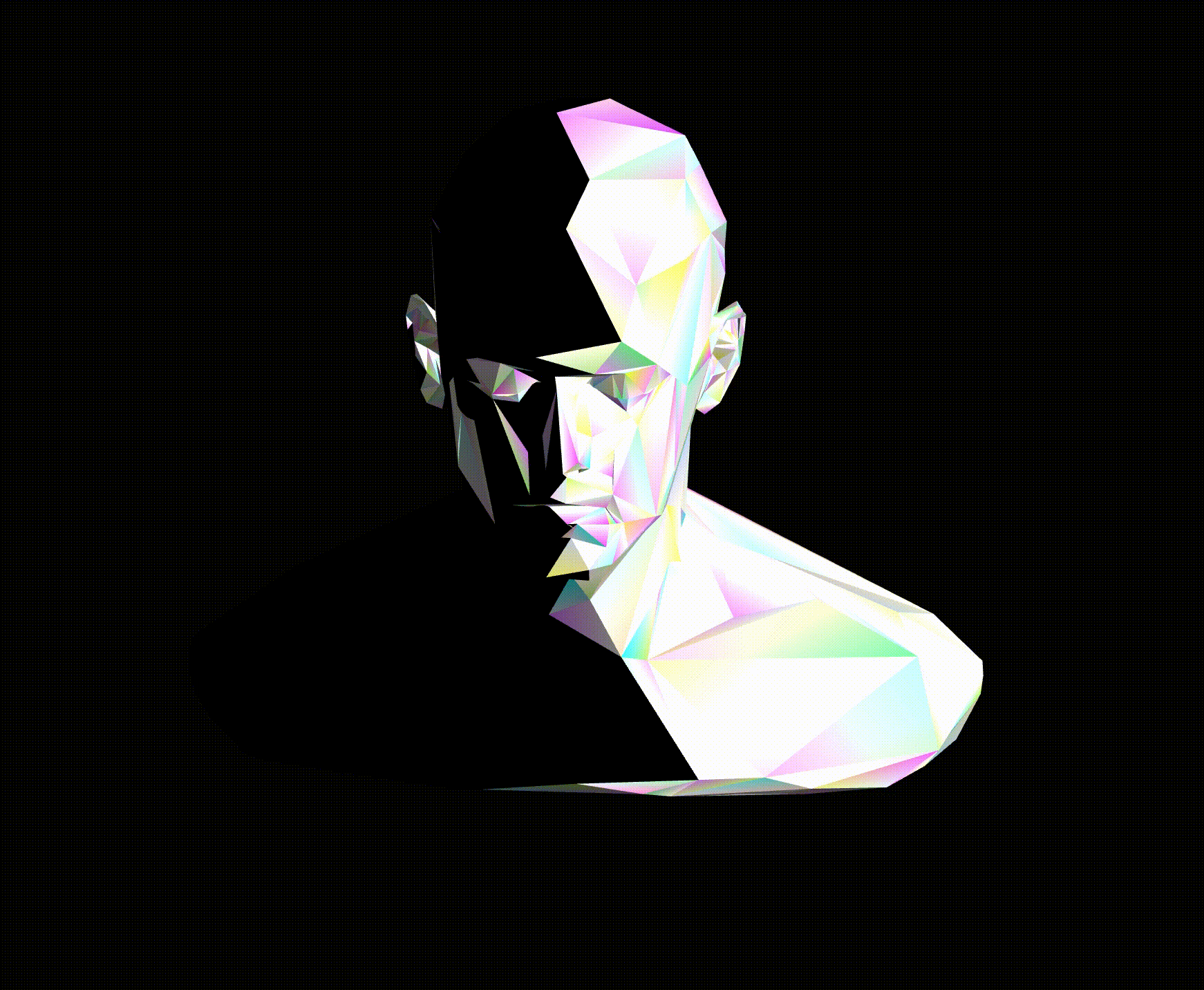## Background

I have been learning Three.js from a lot of examples in its repository. In this time, I pick up two examples and regenerate into new visualization. I am excited to publish my tiny demonstration. Basically, this effect comes from the following images.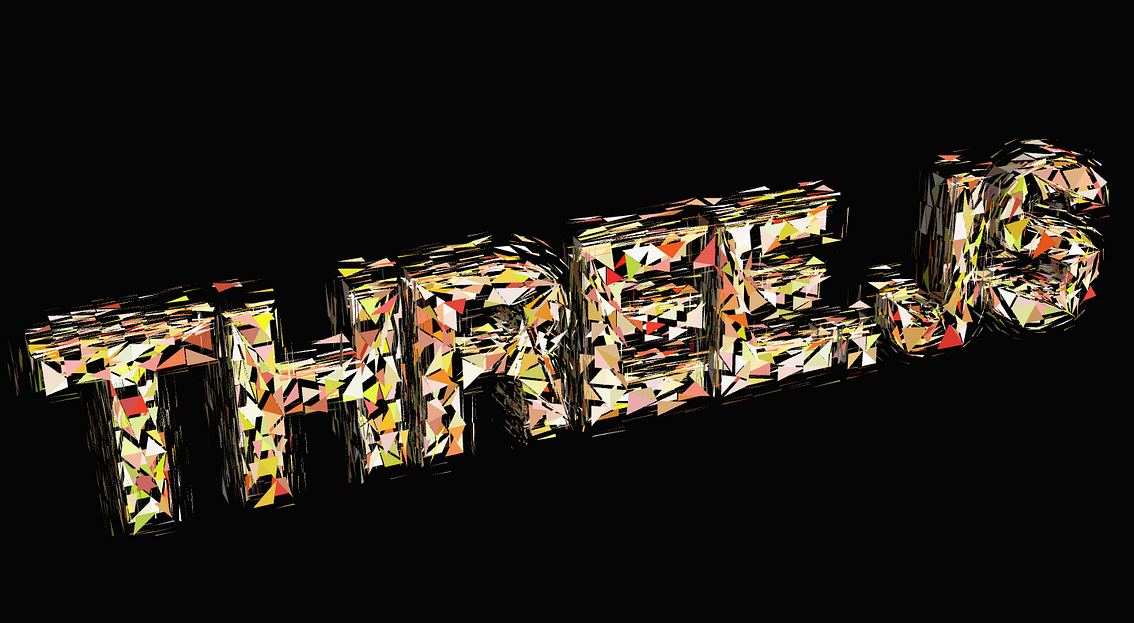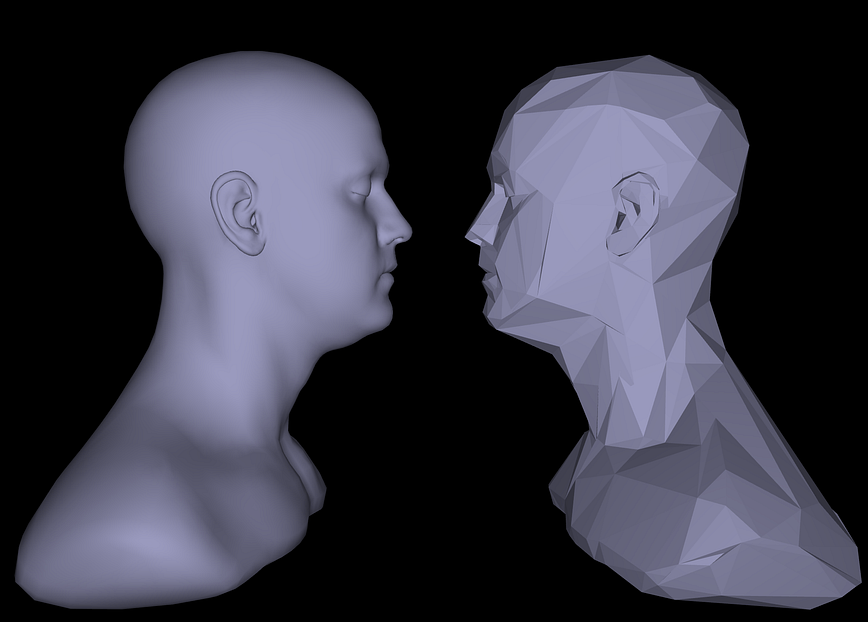#### Workflow

• Create geometry from model
• Create scene, camera
• Create Mesh for polygonal model with shader material
• Render scattering fragments

I place emphasis on the creation of a polygonal object and dismantled triangle parts. Therefore, I will explain these procedures in that point of views next.

## Highlight Code

#### Create polygonal model

In the example of modifier / simplifier , they supply the diminishing code as SimplifyModifier class. It is not native support code in Three.js but well-maintenance code. So we can use it as follows.

var loader = new THREE.FontLoader();
var mesh = gltf.scene.children;
var modifier = new SimplifyModifier();
var simplified = mesh.clone();
simplified.material = simplified.material.clone();
var count = Math.floor(simplified.geometry.attributes.position.count * 0.8); // number of vertices to remove
simplified.geometry = modifier.modify(simplified.geometry, count);
var geometry = new Geometry().fromBufferGeometry(simplified.geometry);
})

We can control how level we abstract the model by the count. Although the variable called simplified is cloned by pure model mesh and the instance type is BufferGeometry, I had to convert it into Geometry for the next process. I don’t know why I should do that but apparently, there is no data for normal in attribute. After converting it to Geometry, recreate as BufferGeometry using fromGeometry method.

geometry = new THREE.BufferGeometry().fromGeometry(geometry);

The difference between the two is as follows.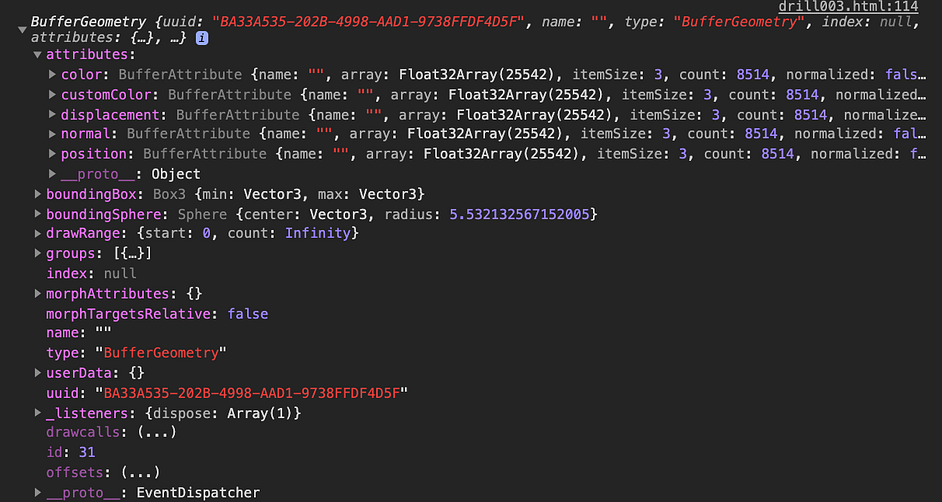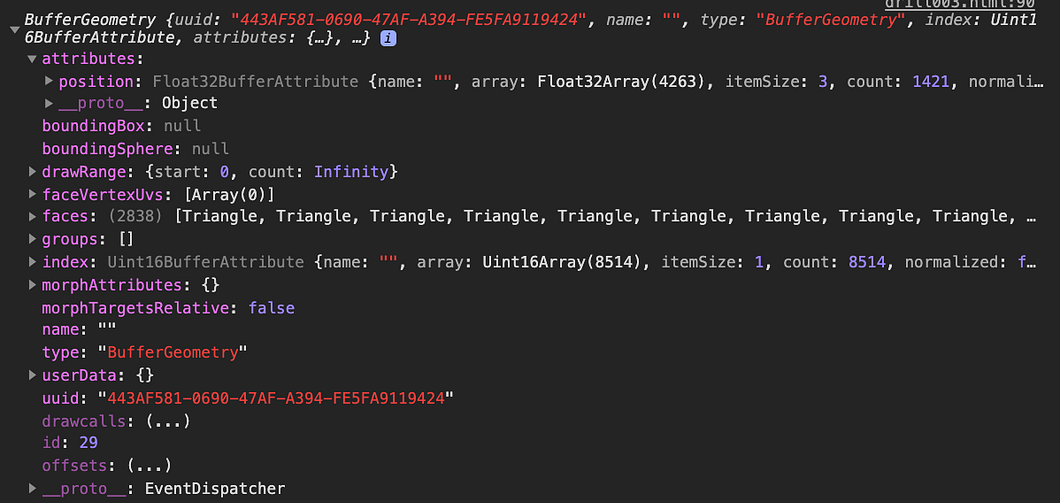## Dispersed triangle parts

To put the triangle faces apart from the 3D model, use a shader material. Before executing shader, set up the number of the fragments first.

var numFaces = Math.floor(geometry.attributes.position.count);
var displacement = new Float32Array(numFaces * 3);
for (var f = 0; f < numFaces; f++) {
var index = 3 * f;
var d = 1000 * (0.5 - Math.random());

for (var i = 0; i < 3; i++) {
displacement[index + (3 * i)] = d;
displacement[index + (3 * i) + 1] = d;
displacement[index + (3 * i) + 2] = d;
}
}

This displacement parameter is used in the shader. What the variable means is how far the triangle parts from original position on animation. The more bigger the value at d parameter is, The more further the distance is. If the value is 0, nothing happens, which means it is not dismantled.

After setting the dismount property, assign it as a attributes in geometry so that the shader can access it.

geometry.setAttribute('displacement', new THREE.BufferAttribute(displacement, 3));

According to amplitude value in the shader, the distance varies. Therefore, I change the value in every 5000 seconds. I introduce Tween to control amplitude value seamlessly.

var isReverse = true
function tween(amplitude, to) {
new TWEEN.Tween(amplitude).to({ value: to, }, 2000)
.onComplete(() => isReverse = !isReverse)
}